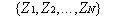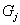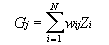# Weighted Averaging

The gridding methods in Surfer use weighted average interpolation algorithms. This means that, with all other factors being equal, the closer a point is to a grid node, the more weight it carries in determining the Z value at that grid node. The difference between gridding methods is how the weighting factors are computed and applied to data points during grid node interpolation.

To understand how weighted averages are applied, consider the equation shown here.

Given N data values:the interpolated value at any grid node (for example,) can be computed as the weighted average of the data values:where

 Gj = interpolated grid node value at node j; N = number of points used to interpolate at each node; Zi Z value at the ithpoint wij weight associated with the ithdata value when computing